# Catch up on the latest AI articles# Time Series Anomaly Detection SOTA Survey

3 main points
✔️ SOTA survey from machine learning and deep learning methods in detecting anomalies in univariate time series data
✔️ Statistical methods are dominant for single and continuous anomalies, while deep learning is dominant for anomalies involving context
✔️ Multivariate and multimodal awaits further investigation

Written by
(Submitted on 1 Apr 2020)
Comments:
Accepted by arXiv.
Subjects: Machine Learning (cs.LG); Machine Learning (stat.ML)code:## first of all

For anomaly detection in time-series data, the 1979 John Tukey in 1979. In addition to statistical methods and machine learning, deep learning methods are now used, and among so many methods, we feel that we need a guideline to choose the best method for each situation.

In this survey paper, we compare the performance of a total of 20 methods from statistical, classical machine learning, and deep learning, with different anomaly detection definitions, datasets, and evaluation metrics, albeit on a univariate basis.

## concept definition

### Outliers and outliers

Outliers and outliers are similar concepts, but there is no consensus on each. Here, we assume that outliers and outliers have the same meaning, as follows.

Outliers: There is a marked deviation from the general data distribution and the outliers form a very small part of the overall data.
(From an academic point of view, this is the only stance that can be taken, but in practice, it is also necessary to separate normal/abnormal values within the main distribution. For example, when multiple events have a causal relationship and the normal/abnormal in the final result must be judged by looking at the variation in intermediate events.)

### Type of Abnormality

The types of anomalies are classified as follows: 1) Point anomalies: A single point deviating from the trend is considered an anomaly. 2) Collective anomalies: Individual values are not anomalous, but a series of values can be considered anomalies. 3) Contextual anomalies: The same value can be regarded as normal or abnormal depending on the situation.

### Stochastic Processes and Time Series

A stochastic process is represented by Z(w, t): w is the sample space and t is a point in time, whereas a time series is a series of observation points measured continuously over time.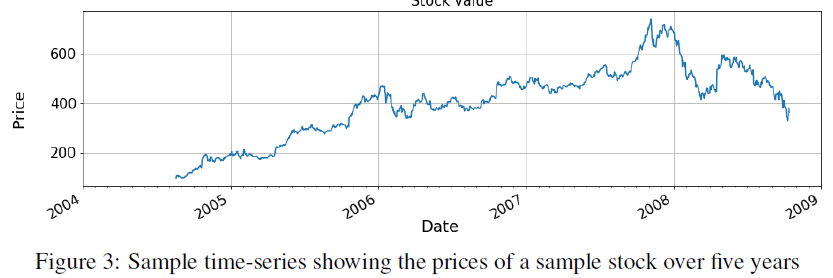### time-series pattern

There are the following patterns in time series data. A trend is a pattern that increases or decreases over time. There are linear trends and non-linear trends. Seasonality is a fluctuation that comes and goes periodically. A cycle is a period that is not fixed and lasts more than one year.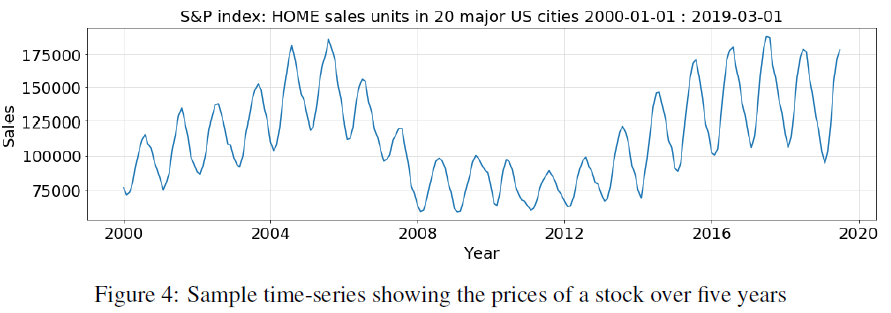The level is the average of the series. The level fluctuates when there is a trend. Stationarity has the same properties (e.g. mean, variance, autocorrelation) for each time interval. White noise is a stochastic process. It is not correlated with time.

### abnormal detection

Anomaly detection of time series data is different from normal/anomalous detection of spatial data in that one data point is considered to have an effect on the next data point and hence sudden changes in the sequence are considered anomalous. Aggarwal classifies anomaly detection into two categories: anomaly detection based on time series prediction and anomaly detection based on the unusual shape of the time series. Most statistical methods use the former, while some machine learning uses time series clustering methods.

Supervised, semi-supervised, unsupervised Abnormality detection

In supervised learning, normal/abnormal labels are assigned to timestamps. In semi-supervised learning, only normal data is used. In unsupervised learning, no labels are used. A widely used method uses the 3σ of the distribution as the criterion for abnormality.

## Selected time series data anomaly detection methods

### Anomaly detection by statistical methods

Autoregressive Model (AR), Moving Average Model (MA), Autoregressive Moving Average Model (ARMA) and ARIMA Model are commonly used for time series analysis. (Commentary)

Simple Exponential Smoothing (SES) was proposed in 1956, so it is an old method, but it has not been mentioned much, so I will explain it a little. In SES, exponential weights are used for nonlinear approximation, while the previous methods use linear approximation.

$$X_{t+1} = \alpha X_t + \alpha (1 - \alpha )X_{t-1} + \alpha (1 - \alpha )^2 X_{t-2} + ... + \alpha (1 - \alpha )^N X_{t-N}$$

$$where \alpha \in [0, 1]$$

Double and Triple Exponential Smoothing (DES, TES) is an extension of SES to model non-stationarity: DES adds another parameter β to smooth the trend, and TES adds another parameter γ to control the seasonality. to control for seasonality.

Time-series Outlier Detection using Prediction Confidence Interval (PCI) is a method published in 2014. It applies a non-linear weighting to the previous data. $$X_t = \frac{\sum_{j=1}^k \omega _ {t - j} X_{t - j}}{\sum_{j=1}^k X_{t - j}}$$.

$$PCI=X_i \pm t_{\alpha , 2k-1} \times s \sqrt{1 + \frac{1}{2k}}$$.

where t is the Student's t-distribution coefficient, s is the standard deviation, and k is the window size.

### Anomaly detection in classical machine learning

K-Means Clustering - Subsequence Time-Series Clustering (STSC) converts time series data into a set of vectors while sliding the window length w by γ, and then applies k-Means to clustering.

Density-Based Spatial Clustering of Applications with Noise (DBSCAN) considers data density in clustering; in 2011, Celik et al. applied it to time series.

Local Outlier Factor (LOF) is a clustering that focuses on k-nearest neighbors and local outliers. In 2015, Oehmcke et al. applied it to time series.

Isolation Forest (iForest) was proposed by Liu et al. in 2008 to separate normal and abnormal in the assembly of Isolation Trees. In the figure below, the anomaly is detected in two separations.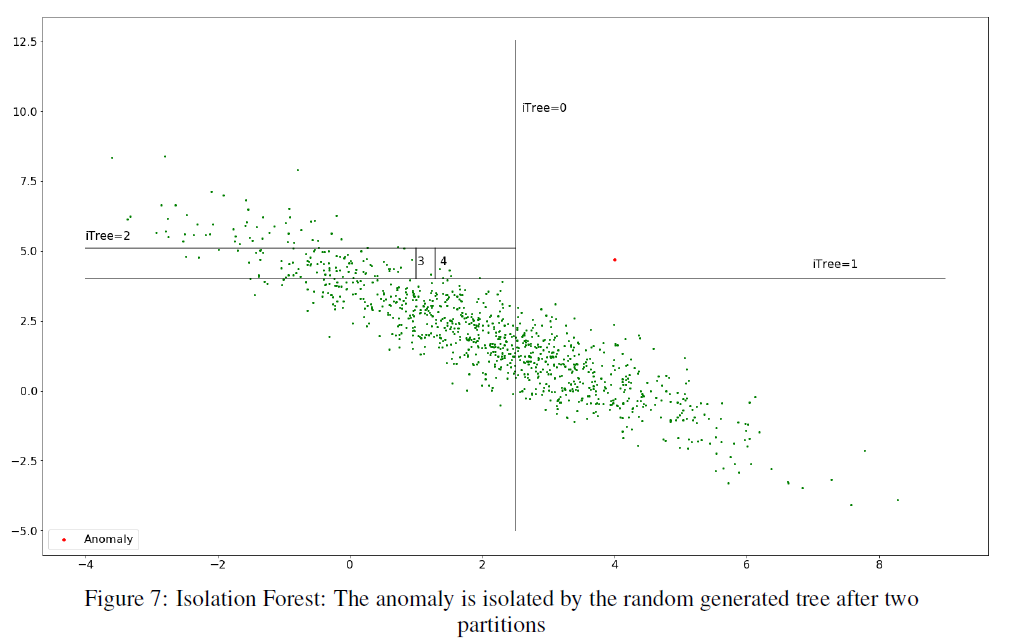One-Class Support Vector Machines (OC-SVM) is semi-supervised learning proposed in 1999 based on SVM. Only normal values are used for training. The time-series data is projected into phase space or cut by a window, vectorized, and then projected into two-dimensional space.

Extreme Gradient boosting (XGBoost, XGB) is a technique often used in Kaggle and KDDCup. Its main feature is scalability. XGBoost is a tree-boosting method, which approximates the time series data by regression to obtain the error function. Since the error function contains some functions that cannot be optimized in Euclidean space, we use Taylor expansion to avoid them.

### Anomaly detection in neural networks

Neural Network Autoregression Model (NNAR), which is based on Multiple Layer Perceptron (MLP), uses lagged data series to create a model that mimics ARIMA. The number of neurons in the input corresponds to the window size.

There has been growing interest in applying Convolutional Neural Networks (CNNs) to time series data in recent years, and Munir et al. have proposed DeepAnT, shown below. A time-series CNN is fed with 1D data. In the individual examples, two sets of Convolution, Max pooling are used, but this structure is optimized for the features of the data set. In our comparative evaluation, we used We also compare the results to those with batch normalization inserted.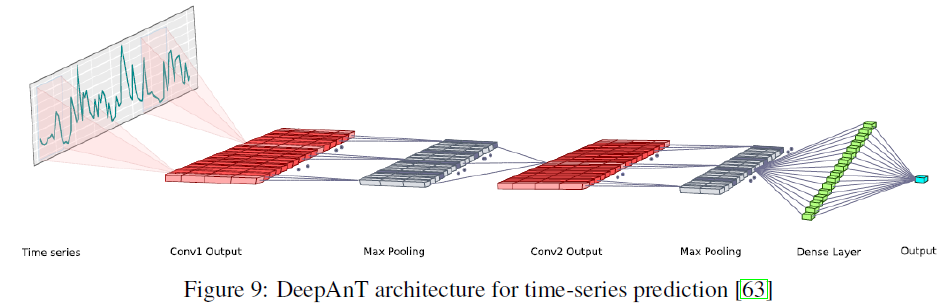Residual Neural Network (Resnet) is a CNN with an additional Residual block, which is applied to time series data by Wang et al. in 2016. It has the problem of overfitting when the number of data is small.
WaveNet has the feature of being able to model a long time with few variables; Borovykh et al. applied it to time series data in 2017.
Long Short Term Memory (LSTM) network,
Gated Recurrent Unit (GRU) is a model originally suitable for long-term data series.

For Autoencoder, Sakurada and Yairi applied it to time series data in 2014. The data is vectorized with window size w and then input into the model. Semi-supervised learning is performed.

## Experiment

### data set

We use the following five datasets: UD is the network traffic data of Yahoo service; UD1 is the raw data; UD2 is randomly added single anomaly points; UD3 is the data with seasonality and randomly added anomaly points; UD4 is the data with change points. processing. NYCT data is the data of taxi use in New York City, including five consecutive anomalies such as NYC Marathon.

### valuation index

We use AUC as the main evaluation index. We also compare the calculation time.

## Result

For Yahoo network access, statistical methods are generally superior for both raw data and synthetic data, such as continuous anomalies, while LSTM and GRU are not so good due to their inappropriate data format.

As for the data on the number of taxis in use, statistical methods have not been able to capture the characteristics well because the situation changes over a long period of time, and machine learning has not done well except for K-means (STSC), while deep learning (especially LSTM, Wavenet) has done well. K-means does not seem to resolve theoretical questions about its application to time-series data, and the results may differ depending on the conditions.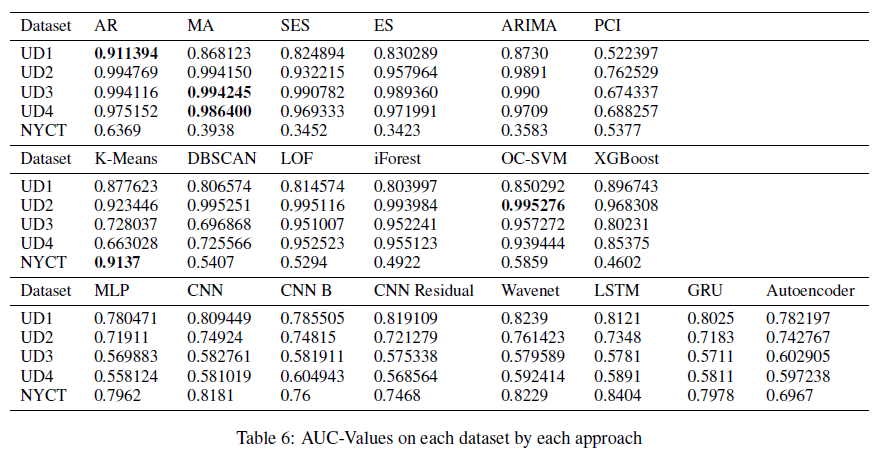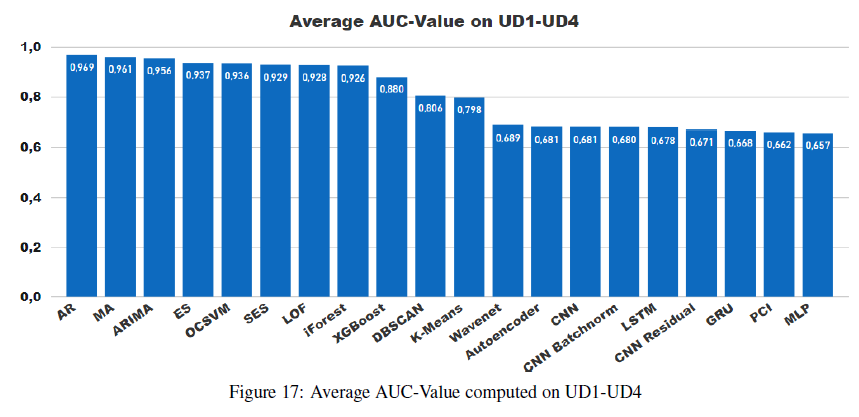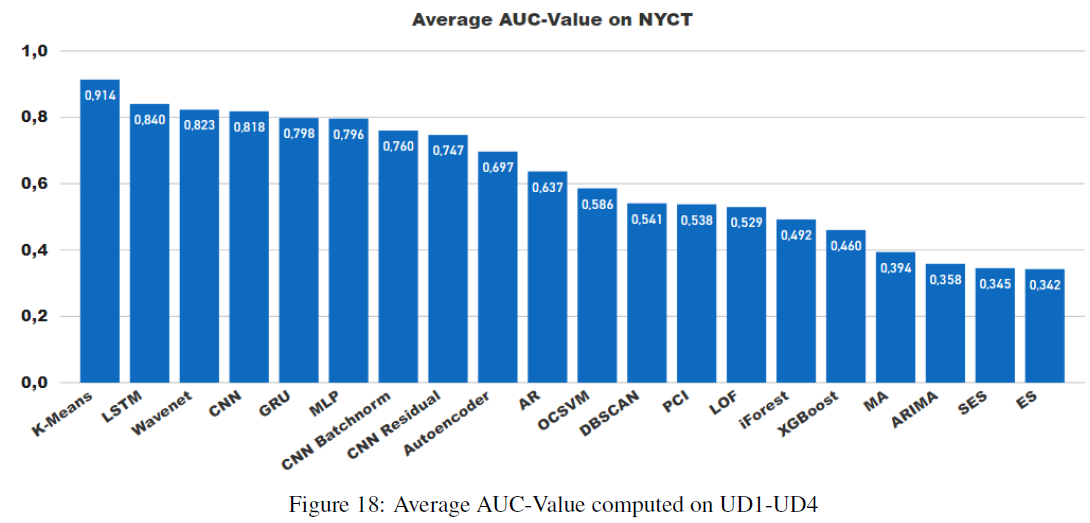(The title of Figure 18 seems to be wrong. The title on the graph is correct.)

On the other hand, statistical methods are still advantageous when it comes to computation time. If you want to perform inference on a real-time system or an edge device, you should also refer to this data. Table 7 shows the total number of 367 models and the computation time per data series by dividing it.